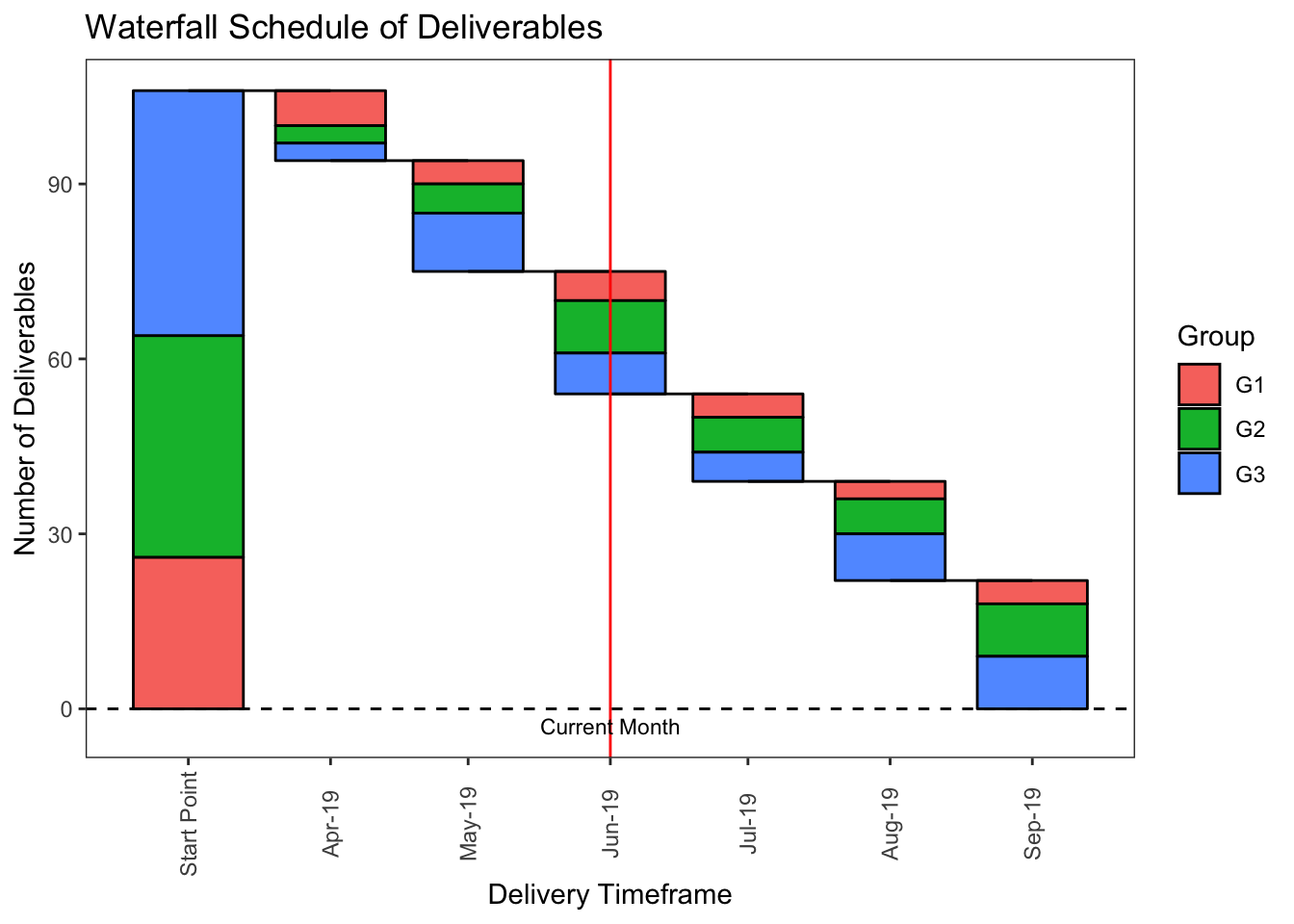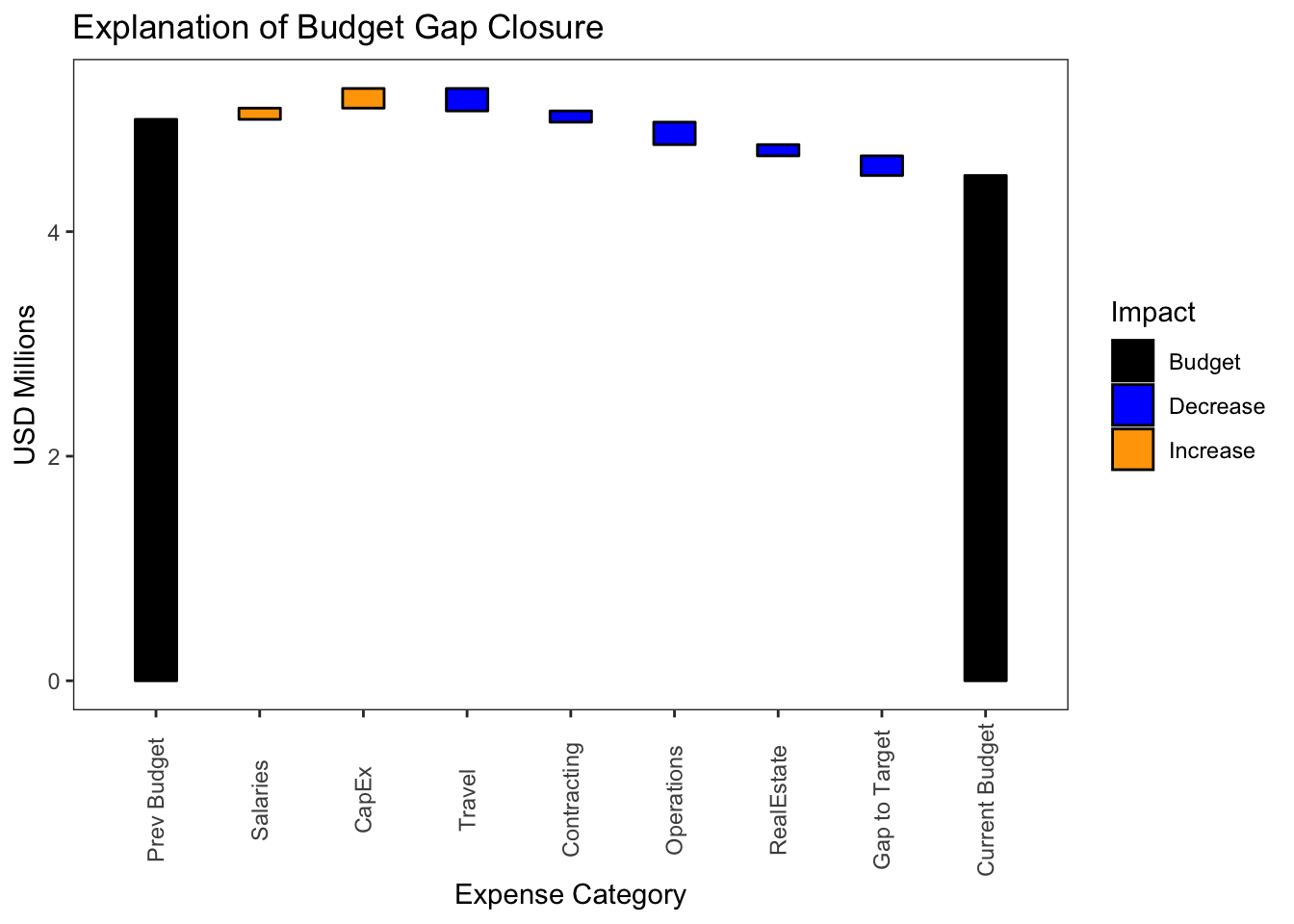# Articles by exploRations in R

October 5, 2021 |

Intro Early last year I posted on my blog an attempt to recreate a Yahoo Finance graphic. Now, thanks to the work of Dr Paul Murrell on the grid and gggrid packages, I have the tools to polish this one off. Reference Article Here is a streamlined ...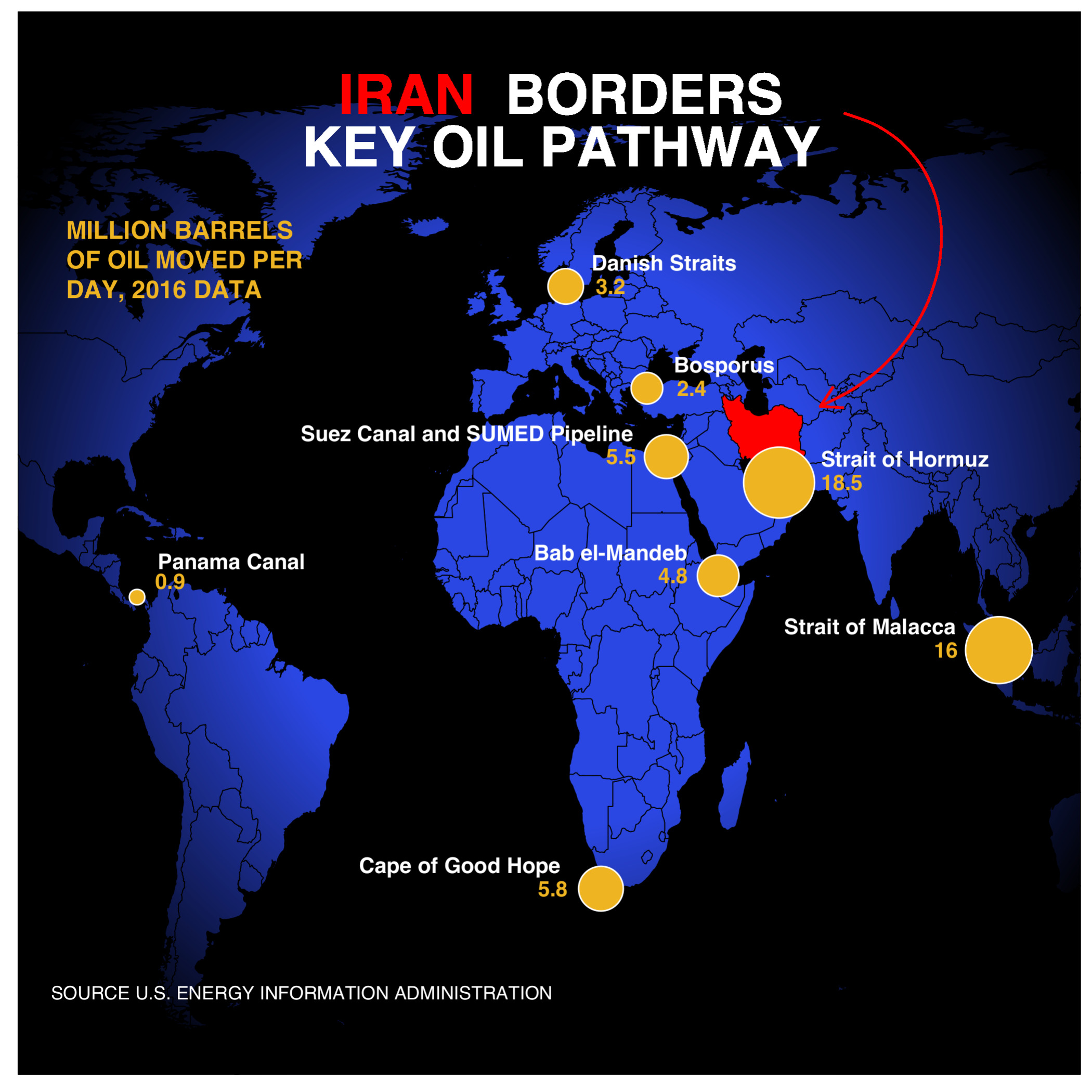### Replicating Plots: Oil Pathways Part 2

October 3, 2021 |

Intro Early last year I posted on my blog an attempt to recreate a Yahoo Finance graphic. Now, thanks to the work of Dr Paul Murrell on the grid and gggrid packages, I have the tools to polish this one off. Reference Article Here is a streamlined ...### Multiple Gauge Plots with Facet Wrap

October 17, 2020 |

Intro Here are some good examples of how to generate gauge plots including multiple gauge plots in R found at stackoverflow. My use case, however, requires the ability to vary the number of plots based on the number of metrics fed into the funct...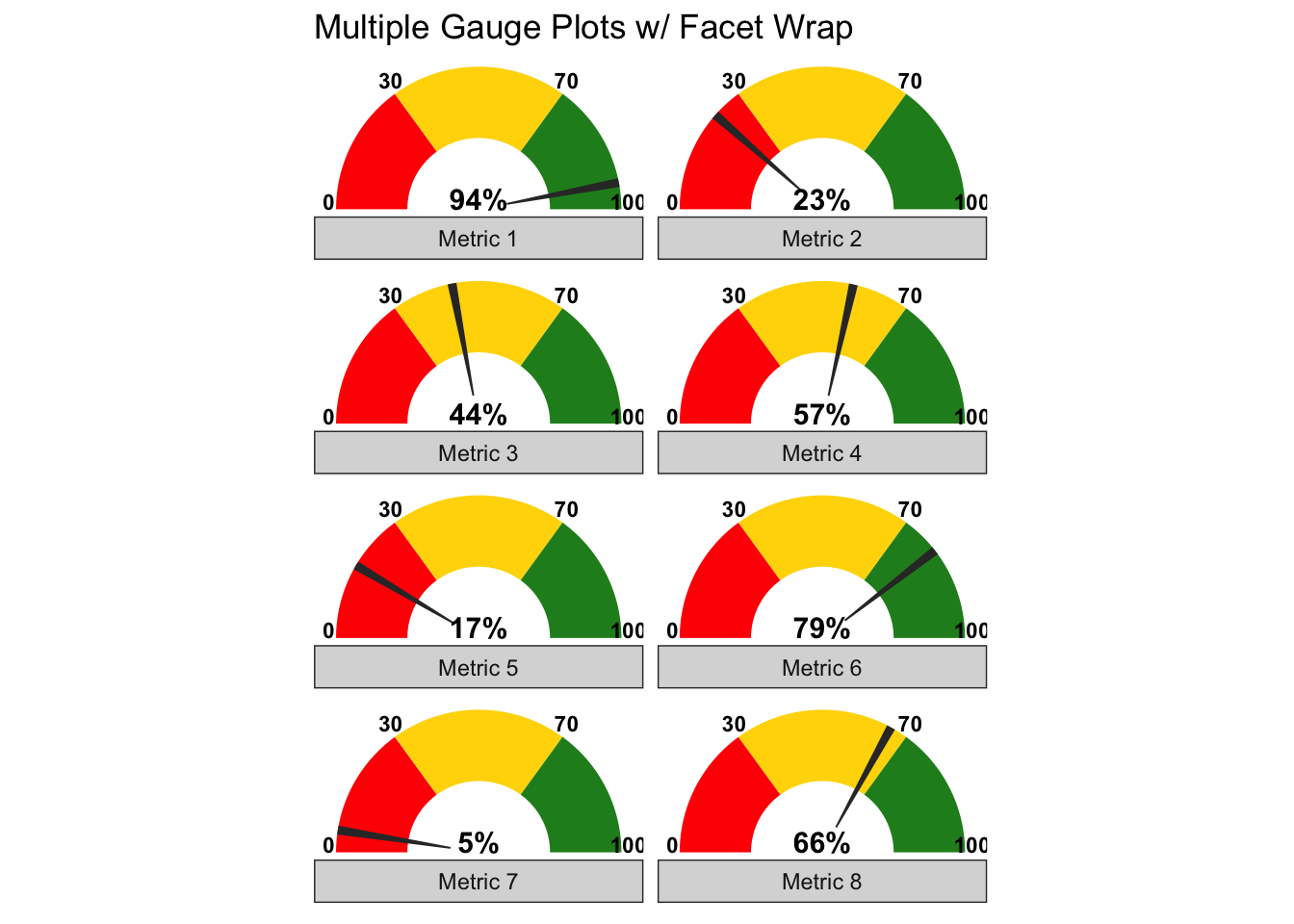### Introducing Boundingbox Package

June 11, 2020 |

`library(boundingbox)`
Intro When we create bounding boxes in images, particularly if we intend to feed them into predictive models for object localization and classification, we are often: Processing many images Classifying the contents of the bounding boxes Standardizing the size of the images Capturing the coordinates The boundingbox package v1.0.1 ...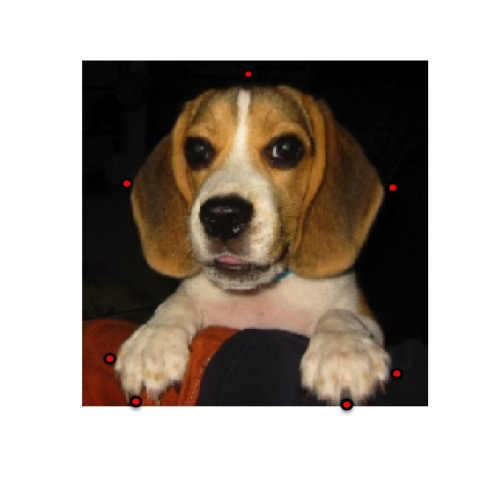### Replicating Plots: Oil Pathways

February 7, 2020 |

Intro I was inspired by this graphic on Yahoo Finance. In the TidyTuesday spirit, I set out to recreate it using ggplot2. There were a few things I would have to figure out - how to apply ggtext to an annotation, how to draw a curved arrow, and how to ...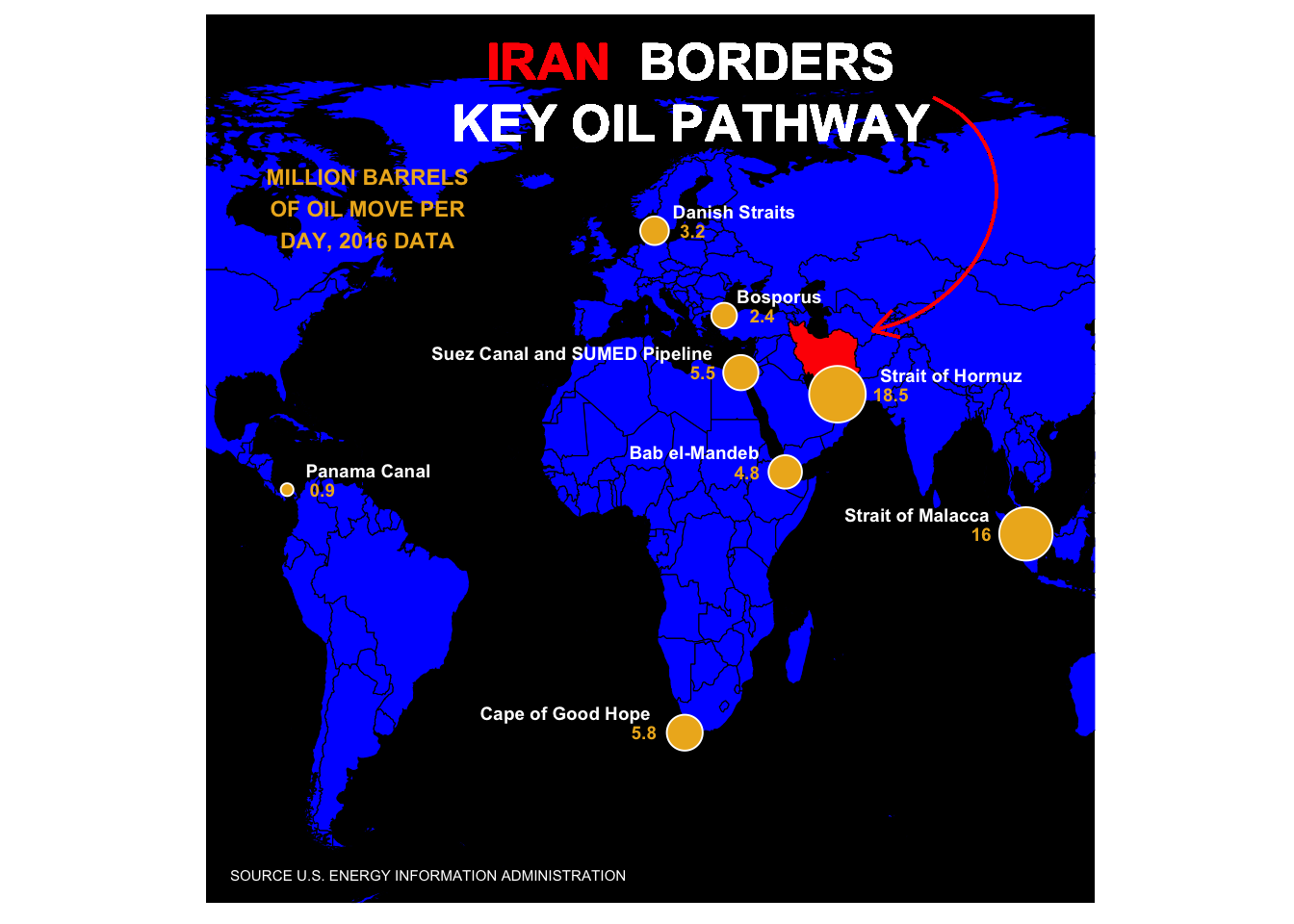### Autumn Barnsley Fern

October 12, 2019 |

Intro I was playing around generating fractals in R when I realized the monochromatic green Barnsely Fern I had on my screen didn’t quite look like the leaves I could see outside my window. It was already Fall. In this post I describe a technique to generate a Barnsley ...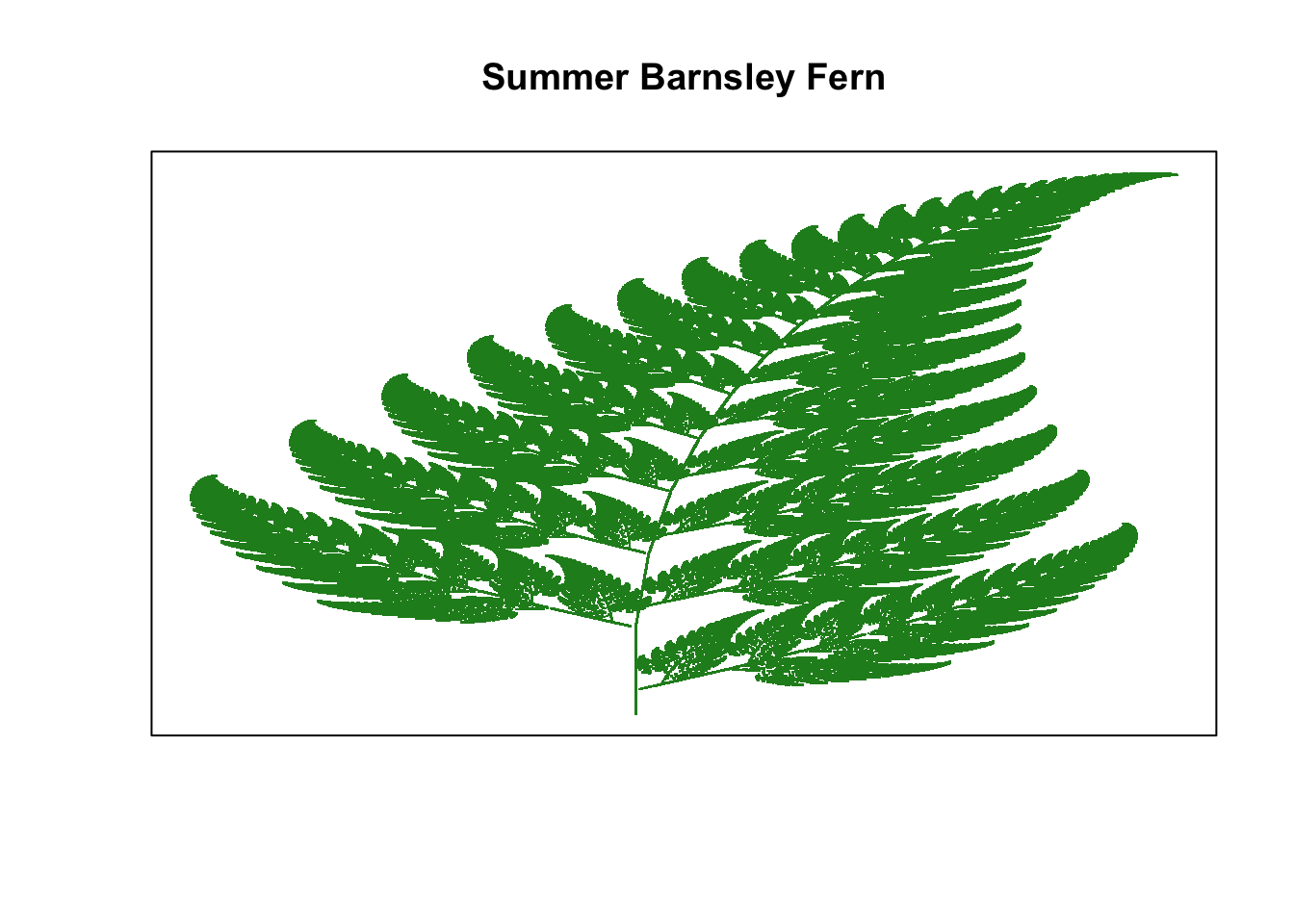### recursive shape fractal generator

August 19, 2019 |

Intro This function allows us to generate the Sierpinski Triangle and explore other recursive shapes with equal length sides following the same algorithm.
```shape <- function(corners, trials = 100000){

corners <- as.integer(corners)
points <- list()

if (corners < 3) stop("Value should be 3 or greater")

for (n in 1:(corners)){
points\$x[n] <- 0 + cos((2*pi*n)/corners)
points\$y[n] <- 0 + sin((2*pi*n)/corners)
}

x <- points\$x
y <- points\$y

trials <- trials
sierpinski <- list()

for (t in 1:trials){
r <- sample(1:corners,1)
x <- (x + points\$x[r]) / sqrt(corners + 1)
y <- (y + points\$y[r]) / sqrt(corners + 1)
sierpinski\$x[t] <- x
sierpinski\$y[t] <- y
}

# I use these colors for random color selection. Update for your own desired selection.
color <- sample(c("royalblue2", "firebrick2", "gold2", "springgreen3", "purple2", "darkorange1"),1)

plot(sierpinski\$x[corners:trials], sierpinski\$y[corners:trials],
xlab = paste0(corners, " Sides Chosen"), ylab = "", xaxt = "n", yaxt = "n", col = color)

}```
When you run the function, you indicate the number of sides for the polygon and adjust the number of trials to change the resolution if desired. ...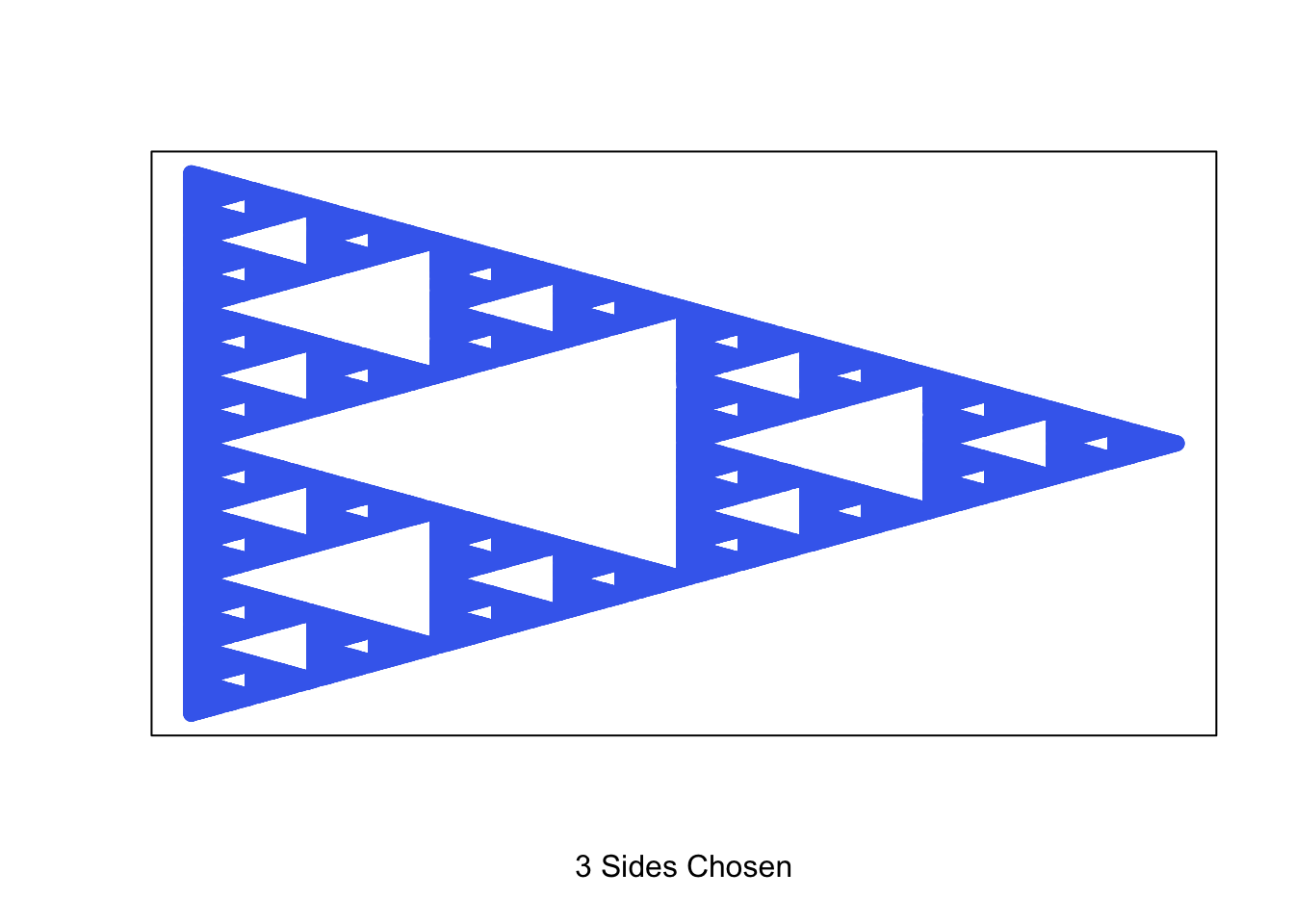### Stacked Waterfall Graphs in R

June 21, 2019 |

Intro In an earlier post I provided a template for creating a basic waterfall graph. Here I introduce a slightly more complex version with stacked bars over a time series. I use dplyr, ggplot2 and lubridate libraries. Stacked Waterfall Graphs This is a version of a waterfall graph I have ...